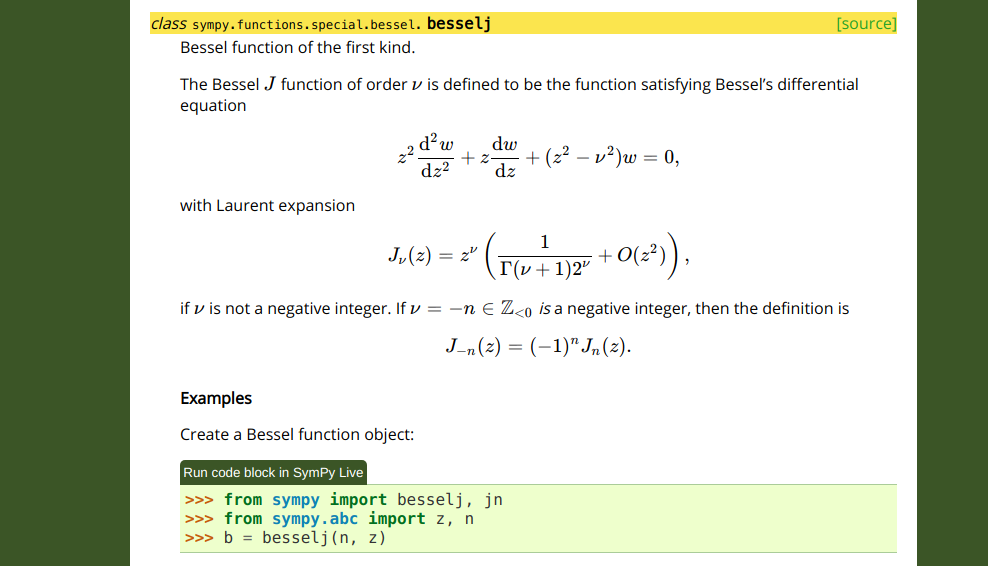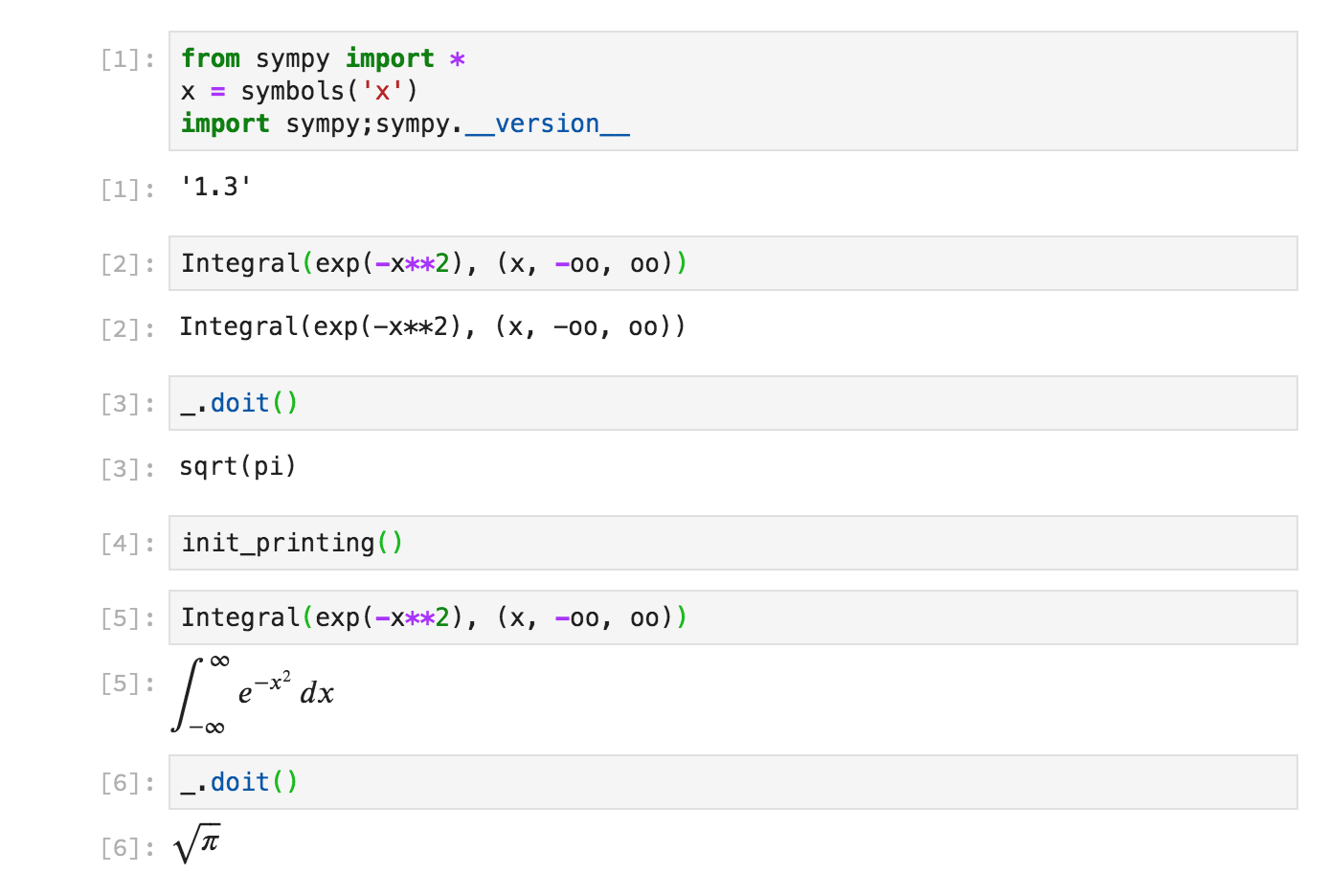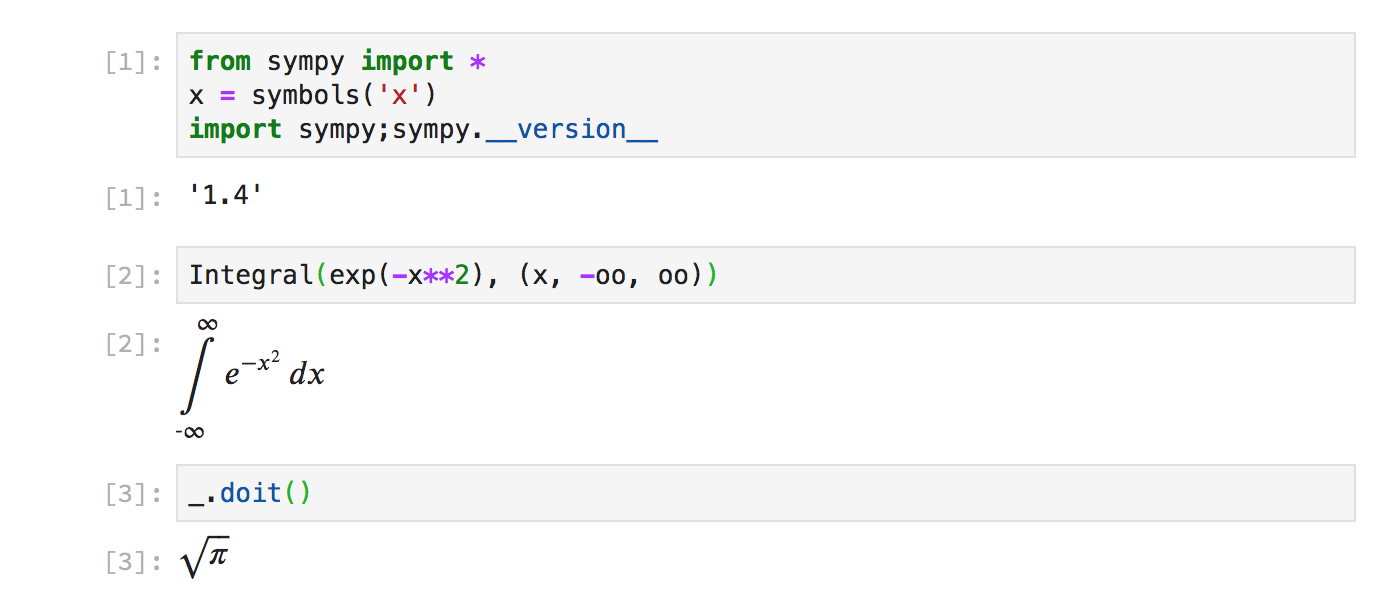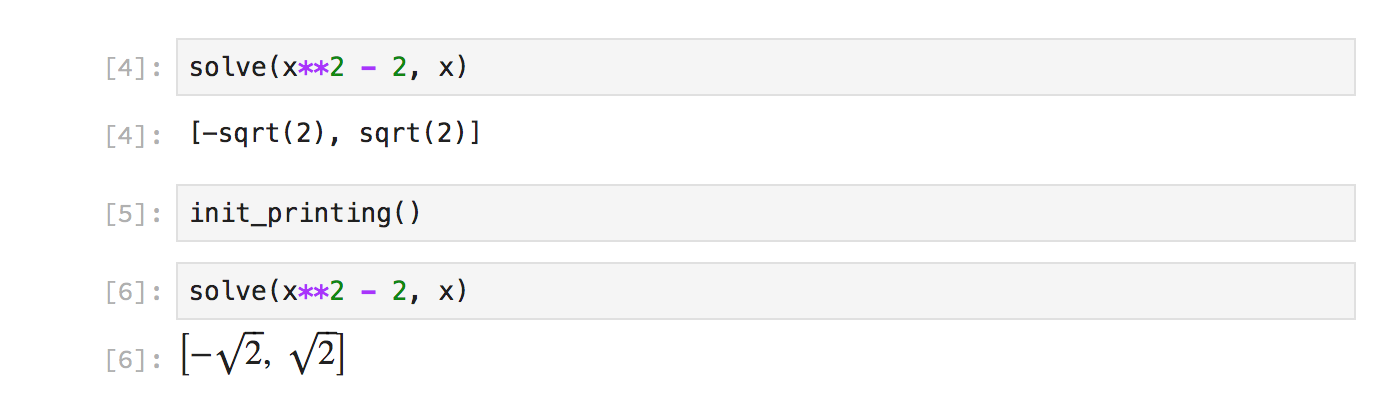# Verifying the Riemann Hypothesis with SymPy and mpmath

Like most people, I've had a lot of free time recently, and I've spent some of it watching various YouTube videos about the Riemann Hypothesis. I've collected the videos I've watched into YouTube playlist. The playlist is sorted with the most mathematically approachable videos first, so even if you haven't studied complex analysis before, you can watch the first few. If you have studied complex analysis, all the videos will be within your reach (none of them are highly technical with proofs). Each video contains parts that aren't in any of the other videos, so you will get something out of watching each of them.

One of the videos near the end of the playlist is a lecture by Keith Conrad. In it, he mentioned a method by which one could go about verifying the Riemann Hypothesis with a computer. I wanted to see if I could do this with SymPy and mpmath. It turns out you can.

## Background Mathematics

$cat mymod/a.py # mymod/a.py from .b import y def func(x): return x + y  The -i flag causes it to edit a.py in-place. Without it, it would just print a diff to the terminal. For implicit star imports and explicit star imports from the same module, removestar works statically, making use of pyflakes. This means none of the code is actually executed. For external imports, it is not possible to work statically as external imports may include C extension modules, so in that case, it imports the names dynamically. removestar can be installed with pip or conda: pip install removestar  or if you use conda conda install -c conda-forge removestar  ### sphinx-math-dollar In SymPy, we make heavy use of LaTeX math in our documentation. For example, in our special functions documentation, most special functions are defined using a LaTeX formula, likeHowever, the source for this math in the docstring of the function uses RST syntax: class besselj(BesselBase): """ Bessel function of the first kind. The Bessel J function of order \nu is defined to be the function satisfying Bessel's differential equation .. math :: z^2 \frac{\mathrm{d}^2 w}{\mathrm{d}z^2} + z \frac{\mathrm{d}w}{\mathrm{d}z} + (z^2 - \nu^2) w = 0, with Laurent expansion .. math :: J_\nu(z) = z^\nu \left(\frac{1}{\Gamma(\nu + 1) 2^\nu} + O(z^2) \right), if :math:\nu is not a negative integer. If :math:\nu=-n \in \mathbb{Z}_{<0} *is* a negative integer, then the definition is .. math :: J_{-n}(z) = (-1)^n J_n(z).  Furthermore, in SymPy's documentation we have configured it so that text between single backticks is rendered as math. This was originally done for convenience, as the alternative way is to write :math:\nu every time you want to use inline math. But this has lead to many people being confused, as they are used to Markdown where single backticks produce code. A better way to write this would be if we could delimit math with dollar signs, like $\nu$. This is how things are done in LaTeX documents, as well as in things like the Jupyter notebook. With the new sphinx-math-dollar Sphinx extension, this is now possible. Writing $\nu$ produces$\nu$, and the above docstring can now be written as class besselj(BesselBase): """ Bessel function of the first kind. The Bessel$J$function of order$\nu$is defined to be the function satisfying Bessel's differential equation .. math :: z^2 \frac{\mathrm{d}^2 w}{\mathrm{d}z^2} + z \frac{\mathrm{d}w}{\mathrm{d}z} + (z^2 - \nu^2) w = 0, with Laurent expansion .. math :: J_\nu(z) = z^\nu \left(\frac{1}{\Gamma(\nu + 1) 2^\nu} + O(z^2) \right), if$\nu$is not a negative integer. If$\nu=-n \in \mathbb{Z}_{<0}$*is* a negative integer, then the definition is .. math :: J_{-n}(z) = (-1)^n J_n(z).  We also plan to add support for $$double dollars$$ for display math so that .. math :: is no longer needed either . For end users, the documentation on docs.sympy.org will continue to render exactly the same, but for developers, it is much easier to read and write. This extension can be easily used in any Sphinx project. Simply install it with pip or conda: pip install sphinx-math-dollar  or conda install -c conda-forge sphinx-math-dollar  Then enable it in your conf.py: extensions = ['sphinx_math_dollar', 'sphinx.ext.mathjax']  ### Google Season of Docs The above work on sphinx-math-dollar is part of work I have been doing to improve the tooling around SymPy's documentation. This has been to assist our technical writer Lauren Glattly, who is working with SymPy for the next three months as part of the new Google Season of Docs program. Lauren's project is to improve the consistency of our docstrings in SymPy. She has already identified many key ways our docstring documentation can be improved, and is currently working on a style guide for writing docstrings. Some of the issues that Lauren has identified require improved tooling around the way the HTML documentation is built to fix. So some other SymPy developers and I have been working on improving this, so that she can focus on the technical writing aspects of our documentation. Lauren has created a draft style guide for documentation at https://github.com/sympy/sympy/wiki/SymPy-Documentation-Style-Guide. Please take a moment to look at it and if you have any feedback on it, comment below or write to the SymPy mailing list. # What's New in SymPy 1.4 This post has been cross-posted on the Quansight Blog. As of November, 2018, I have been working at Quansight. Quansight is a new startup founded by the same people who started Anaconda, which aims to connect companies and open source communities, and offers consulting, training, support and mentoring services. I work under the heading of Quansight Labs. Quansight Labs is a public-benefit division of Quansight. It provides a home for a "PyData Core Team" which consists of developers, community managers, designers, and documentation writers who build open-source technology and grow open-source communities around all aspects of the AI and Data Science workflow. As a part of this, I am able to spend a fraction of my time working on SymPy. SymPy, for those who do not know, is a symbolic mathematics library written in pure Python. I am the lead maintainer of SymPy. SymPy 1.4 was released on April 9, 2019. In this post, I'd like to go over some of the highlights for this release. The full release notes for the release can be found on the SymPy wiki. To update to SymPy 1.4, use conda install sympy  or if you prefer to use pip pip install -U sympy  The SymPy 1.4 release contains over 500 changes from 38 different submodules, so I will not be going over every change, but only a few of the main highlights. A total of 104 people contributed to this release, of whom 66 contributed for the first time for this release. While I did not personally work on any of the changes listed below (my work for this release tended to be more invisible, behind the scenes fixes), I did do the release itself. ## Automatic LaTeX rendering in the Jupyter notebook Prior to SymPy 1.4, SymPy expressions in the notebook rendered by default with their string representation. To get LaTeX output, you had to call init_printing():In SymPy 1.4, SymPy expressions now automatically render as LaTeX in the notebook:However, this only applies automatically if the type of an object is a SymPy expression. For built-in types such as lists or ints, init_printing() is still required to get LaTeX printing. For example, solve() returns a list, so does not render as LaTeX unless init_printing() is called:init_printing() is also still needed if you want to change any of the printing settings, for instance, passing flags to the latex() printer or selecting a different printer. If you want the string form of an expression for copy-pasting, you can use print. ## Improved simplification of relational expressions Simplification of relational and piecewise expressions has been improved: >>> x, y, z, w = symbols('x y z w') >>> init_printing() >>> expr = And(Eq(x,y), x >= y, w < y, y >= z, z < y) >>> expr x = y ∧ x ≥ y ∧ y ≥ z ∧ w < y ∧ z < y >>> simplify(expr) x = y ∧ y > Max(w, z)  >>> expr = Piecewise((x*y, And(x >= y, Eq(y, 0))), (x - 1, Eq(x, 1)), (0, True)) >>> expr ⎧ x⋅y for y = 0 ∧ x ≥ y ⎪ ⎨x - 1 for x = 1 ⎪ ⎩ 0 otherwise >>> simplify(expr) 0  ## Improved MathML printing The MathML presentation printer has been greatly improved, putting it on par with the existing Unicode and LaTeX pretty printers. >>> mathml(Integral(exp(-x**2), (x, -oo, oo)), 'presentation') <mrow><msubsup><mo>&#x222B;</mo><mrow><mo>-</mo><mi>&#x221E;</mi></mrow><mi>&#x221E;</mi></msubsup><msup><mi>&ExponentialE;</mi><mrow><mo>-</mo><msup><mi>x</mi><mn>2</mn></msup></mrow></msup><mo>&dd;</mo><mi>x</mi></mrow>  If your browser supports MathML (at the time of writing, only Firefox and Safari), you should see the above presentation form for Integral(exp(-x**2), (x, -oo, oo)) below: $∫-∞∞ⅇ-x2ⅆx$ ## Improvements to solvers Several improvements have been made to the solvers. >>> eq = Eq((x**2 - 7*x + 11)**(x**2 - 13*x + 42), 1) >>> eq 2 x - 13⋅x + 42 ⎛ 2 ⎞ ⎝x - 7⋅x + 11⎠ = 1 >>> solve(eq, x) # In SymPy 1.3, this only gave the partial solution [2, 5, 6, 7] [2, 3, 4, 5, 6, 7]  The ODE solver, dsolve, has also seen some improvements. Two new hints have been added. 'nth_algebraic' solves ODEs using solve by inverting the derivatives algebraically: >>> f = Function('f') >>> eq = Eq(f(x) * (f(x).diff(x)**2 - 1), 0) >>> eq ⎛ 2 ⎞ ⎜⎛d ⎞ ⎟ ⎜⎜──(f(x))⎟ - 1⎟⋅f(x) = 0 ⎝⎝dx ⎠ ⎠ >>> dsolve(eq, f(x)) # In SymPy 1.3, this only gave the solution f(x) = C1 - x [f(x) = 0, f(x) = C₁ - x, f(x) = C₁ + x]  'nth_order_reducible' solves ODEs that only involve derivatives of f(x), via the substitution$g(x)=f^{(n)}(x)\$.

>>> eq = Eq(Derivative(f(x), (x, 2)) + x*Derivative(f(x), x), x)
>>> eq
2
d           d
x⋅──(f(x)) + ───(f(x)) = x
dx           2
dx
>>> dsolve(eq, f(x))
⎛√2⋅x⎞
f(x) = C₁ + C₂⋅erf⎜────⎟ + x
⎝ 2  ⎠


## Dropping Python 3.4 support

This is the last release of SymPy to support Python 3.4. SymPy 1.4 supports Python 2.7, 3.4, 3.5, 3.6, 3.7, and PyPy. What's perhaps more exciting is that the next release of SymPy, 1.5, which will be released later this year, will be the last version to support Python 2.7.

Our policy is to drop support for major Python versions when they reach their End of Life. In other words, they receive no further support from the core Python team. Python 3.4 reached its end of life on May 19 of this year, and Python 2.7 will reach its end of life on January 1, 2020.

I have blogged in the past on why I believe it is important for library authors to be proactive in dropping Python 2 support, and since then a large number of Python libraries have either dropped support or announced their plans to by 2020.

Having Python 2 support removed will not only allow us to remove a large amount of compatibility cruft from our codebase, it will also allow us to use some Python 3-only features that will clean up our API, such as keyword-only arguments, type hints, and Unicode variable names. It will also enable several internal changes that will not be visible to end-users, but which will result in a much cleaner and more maintainable codebase.

If you are still using Python 2, I strongly recommend switching to Python 3, as otherwise the entire ecosystem of Python libraries is soon going to stop improving for you. Python 3 is already highly recommended for SymPy usage due to several key improvements. In particular, in Python 3, division of two Python ints like 1/2 produces the float 0.5. In Python 2, it does integer division (producing 1/2 == 0). The Python 2 integer division behavior can lead to very surprising results when using SymPy (imagine writing x**2 + 1/2*x + 2 and having the x term "disappear"). When using SymPy, we recommend using rational numbers (like Rational(1, 2)) and avoiding int/int, but the Python 3 behavior will at least maintain a mathematically correct result if you do not do this. SymPy is also already faster in Python 3 due to things like math.gcd and functools.lru_cache being written in C, and general performance improvements in the interpreter itself.

## And much more

These are only a few of the highlights of the hundreds of changes in this release. The full release notes can be found on our wiki. The wiki also has the in progress changes for our next release, SymPy 1.5, which will be released later this year. Our bot automatically collects release notes from every pull request, meaning SymPy releases have very comprehensive and readable release notes pages. If you see any mistakes on either page, feel free to edit the wiki and fix them.